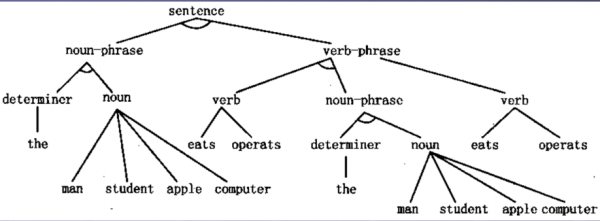## Part 1 形式语言与文法

（一）文法与自动机

• V：变量/非终极符号的非空有穷集；
• T：终极符的非空有穷集，V∩T=Ø；
• P：产生式的非空有穷集合；
• S：开始符号，S是V中的元素。

P是生成式的有穷集合，生成式的基本形式是α→β，这里α和β都是（V∪T）中的元素，即它们都是由变元和终结符组成的符号串，但要求α至少含有一个非终结符；在形式文法定义中生成式集合P是至关重要的，它决定了语言是如何构造出来的。

0型文法，又称为短语文法。这种文法对生成式α→β不作特殊限制，α和β可以是任意的文法符号串，当然α不能是空字符串；0型文法是形式语言谱系中最大的文法类；由0型文法产生的形式语言恰是图灵机所识别的语言类，即递归可枚举语言。

1型文法，又称为上下文有关文法；这种文法要求生成式α→β满足|α|≤|β|，即β要至少和α一样长；由1型文法产生的语言称为1型语言或上下文有关语言；1型语言恰是非确定型线性有界自动机所识别的语言类。

2型文法，又称为上下文无关文法；这种文法要求生成式α→β中的α必须是变元；由2型文法产生的语言称为2型语言或上下文无关语言；2型语言恰是由下推自动机所识别的语言类。

3型文法，又称为正则文法；这种文法分为两种类型：生成式的形式必须是A→ωB或A→ω，其中A，B都是变元，ω是终结符串（可以是空串），这种特殊的正则文法称为右线性文法；第二类正则文法称为左线性文法，它要求生成式必须是A→Bω，或A→ω的形式。由正则文法生成的语言称为正则语言，它恰是有穷自动机所识别的语言类。

(1)理解语句中的每一个词。

(2)以这些词为基础组成一个可以表达整个语句意义的结构。

• (1)语法分析。将单词之间的线性次序变换成一个显示单词如何与其他单词相关联的结构。语法分析确定语句是否合乎语法，因为一个不合语法的语句就更难理解。
• (2)语义分析。各种意义被赋予由语法分析程序所建立的结构，即在语法结构和任务领域内对象之间进行映射变换。
• (3)语用分析。为确定真正含义，对表达的结构重新加以解释。

（二）语义和语用

``````<sentence>∷=<noun-phrase><verbphrase>
<noun-phrase>∷=<determiner><noun>
<verb-phrase>∷=<verb><nounphrase>|<verb>
<determiner>∷=the|a|an
<noun>∷=man|student|apple|computer
<verb>∷=eats|operats``````

``````S→PRESENT the
ATTRIBUTE of SHIP
PRESENT→what is|can
you tell me
ATTRIBUTE→length|class
SHIP→the
SHIPNAME|CLASSNAME class ship
SHIPNAME→Kunming|Liaoning
CLASSNAME→destroyer|aircraft
carrier``````

(1)相同的事物，例如：“小华有个计算器，小刘想用它。”单词“它”和“计算器”指的是同一物体。

(2)事物的一部分，例如：“小林穿上她刚买的大衣，发现掉了一个扣子。”“扣子”指的是“刚买的大衣”的一部分。

(3)行动的一部分，例如：“王宏去北京出差，他乘早班飞机动身。”

(4)与行动有关的事物，例如：“李明准备骑车去上学，但他骑上车子时，发现车胎没气了。”李明的自行车应理解为是与他骑车去上学这一行动有关的事物。

(5)因果关系，例如：“今天下雨，所以不能上早操。”下雨应理解为是不能上操的原因。

(6)计划次序，例如：“小张准备结婚，他决定再找一份工作干。”

## Part 2 语言学与计算机科学：从字符串匹配到元胞自动机

（一）KMP算法

KMP算法于1977年由Knuth（TAOCP作者）, Morris和Pratt提出，将字符串匹配的时间复杂度降至O（m+n），其基本思路就是：假设现在文本串s匹配到 i 位置，模式串t匹配到 j 位置，如果j = -1，或者当前字符匹配成功（即S[i] == P[j]），都令i++，j++，继续匹配下一个字符；如果j != -1，且当前字符匹配失败（即S[i] != P[j]），则令 i 不变，j = next[j]。此举意味着失配时，模式串t相对于文本串s向右移动了j - next [j] 位。换言之，当匹配失败时，模式串向右移动的位数=失配字符所在位置 - 失配字符对应的next 值，即移动的实际位数为j，且此值大于等于1。

next 数组各值的含义：代表当前字符之前的字符串中，有多大长度的相同前缀后缀。例如如果next [j] = k，代表j 之前的字符串中有最大长度为k 的相同前缀后缀。这也意味着在某个字符失配时，该字符对应的next 值会告诉你下一步匹配中，模式串应该跳到哪个位置（跳到next [j] 的位置）。如果next [j] 等于0或-1，则跳到模式串的开头字符，若next [j] = k 且 k > 0，代表下次匹配跳到j 之前的某个字符，而不是跳到开头，且具体跳过了k 个字符。

KMP的next 数组相当于告诉我们：当模式串中的某个字符跟文本串中的某个字符匹配失配时，模式串下一步应该跳到哪个位置。如模式串中在j 处的字符跟文本串在i 处的字符匹配失配时，下一步用next [j] 处的字符继续跟文本串i 处的字符匹配，相当于模式串向右移动 j - next[j] 位。

C++代码如下：

``````void getNext(string s, int *next){
int n = s.length(), i = 0, j = -1;
next = -1;
while (i < n - 1)
{
if (j == -1 || s[i] == s[j])
next[++i] = ++j;
else
j = next[j];
}
}

int kmp(string s, string t){
int n1 = s.length(), n2 = t.length(), i = 0, j = 0;
int *next = new int[n2];
getNext(t, next);
while (i<n1&&j<n2){
if (j == -1 || s[i] == t[j]){
i++;
j++;
}
else{
j = next[j];
}
}
if (j==n2){
return i - j + 1;
}
else{
return 0;
}
}
``````

（二） TRIE树与AC自动机

Trie树又名字典树，从字面意思即可理解，这种树的结构像英文字典一样，相邻的单词一般前缀相同，之所以时间复杂度低，是因为其采用了以空间换取时间的策略。将每个字符串插入到trie树中，到达特定的结尾节点时，在这个节点上进行标记，通过前序遍历此树，即可得到字符串从小到大的顺序。

``````class Node{
public:
int count; //记录该处字符串个数
Node* char_arr[NUM];  //分支
char* current_str;   //记录到达此处的路径上的所有字母组成的字符串
Node();//构造函数略,下同
};

class Trie{
public:
Node* root;
Trie();

void insert(char* str){
int i = 0;
Node* parent = root;
while(str[i] != '\0'){
if(parent->char_arr[str[i] - 'a'] == NULL){
parent->char_arr[str[i] - 'a'] = new Node();
strcat(parent->char_arr[str[i] - 'a']->current_str, parent->current_str);
char str_tmp;
str_tmp = str[i];
str_tmp = '\0';
strcat(parent->char_arr[str[i] - 'a']->current_str, str_tmp);
parent = parent->char_arr[str[i] - 'a'];
}
else{
parent = parent->char_arr[str[i] - 'a'];
}
i++;
}
parent->count++;
}

void output(Node* &node, char** str, int& count){
if(node != NULL){
if(node->count != 0){
for(int i = 0; i < node->count; i++){
str[count++] = node->current_str;
}
for(int i = 0; i < NUM; i++){
output(node->char_arr[i], str, count);
}
}
}
}
};
``````

AC（Aho-Corasick）自动机于1975年由贝尔实验室的A.Aho（《龙书》作者）和M.Corasick提出，可以看成是kmp在多字符串情况下扩展形式，可以用来处理多模式串匹配。只要为这些模式串建立一个trie树，然后再为每个节点建立一个失败指针，也就是类似与kmp的next函数，让我们知道如果匹配失败，可以再从哪个位置重新开始匹配。

``````struct Trie {
int next;
int fail, flag;
void init ()
{
memset(next, 0, sizeof(next));
fail = -1;
flag = 0;
}
} trie[MAXM];

void make_ac_automation() {
int h = 0, t = 0;
q[t++] = 0;
while(h < t)
{
int u = q[h++], v, j;
for(int i = 0; i < 4; i++)
{
if(trie[u].next[i])
{
v = trie[u].next[i];
j = trie[u].fail;
while(j != -1 && !trie[j].next[i]) j = trie[j].fail;
if(j == -1) trie[v].fail = 0;
else
{
trie[v].fail = trie[j].next[i];
trie[v].flag |= trie[trie[v].fail].flag;
}
q[t++] = v;
}
else
{
j = trie[u].fail;
while(j != -1 && !trie[j].next[i]) j = trie[j].fail;
if(j == -1) trie[u].next[i] = 0;
else trie[u].next[i] = trie[j].next[i];
}
}
}
}
``````

（三）TRIE图

Trie图实际上是一个确定性自动机，比AC自动机增加了确定性这个属性，对于AC自动机来说，当碰到一个不匹配的节点后可能要进行好几次回溯才能进行下一次匹配。但是对于trie图来说，可以每一步进行一次匹配，每碰到一个输入字符都有一个确定的状态节点。trie图的后缀节点跟ac自动机的后缀指针基本一致，区别在于trie图的根添加了了所有字符集的边。另外trie图还会为每个节点补上所有字符集中的字符的边，而这个补边的过程实际上也是一个求节点的后缀节点的过程，不过这些节点都是虚的，我们不把它们加到图中，而是找到它们的等价节点即它们的后缀节点，从而让这些边指向后缀节点就可以了。

Trie图主要利用两个概念实现这种目的。一个是后缀节点，也就是每个节点的路径字符串去掉第一个字符后的字符串对应的节点。计算这个节点的方法，是通过它父亲节点的后缀节点，很明显它父亲的后缀节点与它的后缀节点的区别就是还少一个尾字符，设为c。所以节点的父节点的指针的c孩子就是该节点的后缀节点。但是因为有时候它父亲不一定有c孩子，所以还得找一个与父亲的c孩子等价的节点。于是就碰到一个寻找等价节点的问题。

``````bool IsMatch(string str)  {
int len = str.length();
Node* tmp = root;
for (int i = 0; i < len; i++) {
while (tmp != root && tmp->kids[str[i] - 'a'] == NULL)
tmp = tmp->fail;
if (tmp->kids[str[i] - 'a'] != NULL)
tmp = tmp->kids[str[i] - 'a'];
else
tmp = root;
if (tmp->isEnd == true)
return true;
}
return false;
}
``````

（四）DFA与NFA

（1） K是一个有穷非空集，集合中的每个元素称为一个状态；

（2） ∑是一个有穷字母表，∑中的每个元素称为一个输入符号；

（3） F是一个从K×∑→K的单值转换函数，即F（R，a）＝Q，（R，Q∈K）表示当前状态为R，如果输入字符a，则转到状态Q，状态Q称为状态R的后继状态；

（4） S∈K，是惟一的初态；

（5） Z是K的子集，是一个终态集。

（1） k是一个有穷非空集，集合中的每个元素称为一个状态；

（2） ∑是一个有穷字母表，∑中的每个元素称为一个输入符号；

（3） F是一个从K×∑→K的子集的转换函数；

（4） SK，是一个非空的初态集；

（5） ZK，是一个终态集。

（1）NFA的初始状态S为一个状态集，即允许有多个初始状态；

（2）NFA中允许状态在某输出边上有相同的符号，即对同一个输入符号可以有多个后继状态。即DFA中的F是单值函数，而NFA中的F是多值函数。

（1） 设DFA的状态集K中有一状态为S(i_to_j)（Si到Sj所有元素的集合，是S的子集），若对某符号a∈∑，在NFA中有F{[S(i_to_j) ]，a}=S′(i_to_k)，则令F为DFA的一个转换函数。若S′(i_to_k)不在K中，则将其作为新的状态加入到K中。

（2） 重复第2步，直到K中不再有新的状态加入为止。

（3） 上面得到的所有状态构成DFA的状态集K，转换函数构成DFA的F，DFA的字母表仍然是NFA的字母表∑。

（4） DFA中凡是含有NFA终态的状态都是DFA的终态。

（1） 若Q∈I，则Q∈ε-closure（I）；

（2） 若Q∈I，则从Q出发经过任意条ε弧而能到达的任何状态Q’，则Q’∈ε-closure（I）。

NFA确定化的实质是以原有状态集上的子集作为DFA上的一个状态，将原状态间的转换为该子集间的转换，从而把不确定有限自动机确定化。经过确定化后，状态数可能增加，而且可能出现一些等价状态，这时就需要简化。

``````string NODE; //结点集合
string CHANGE; //终结符集合
int N;    //NFA边数

struct edge{
string first;
string change;
string last;
}edge;

struct my_change{
string ltab;
string myset[MAXS];
}my_change;

void my_sort(string &a) {
int i,j;
char b;
for(j=0;j<a.length();j++){
for(i=0;i<a.length();i++){
if(NODE.find(a[i])>NODE.find(a[i+1])) {
b=a[i];
a[i]=a[i+1];
a[i+1]=b;
}
}
}
}

void eclousure(char c,string &he,edge b[]) {
int k;
for(k=0;k<N;k++) {
if(c==b[k].first){
if(b[k].change=="*") {
if(he.find(b[k].last)>he.length()){
he+=b[k].last;
eclousure(b[k].last,he,b);
}
}
}
}
}

void move(change &he,int m,edge b[]) {
int i,j,k,l;
k=he.ltab.length();
l=he.myset[m].length();

for(i=0;i<k;i++){
for(j=0;j<N;j++){
if((CHANGE[m]==b[j].change)&&(he.ltab[i]==b[j].first)){
if(he.myset[m].find(b[j].last)>he.myset[m].length()){
he.myset[m]+=b[j].last;
}
}
}
}

for(i=0;i<l;i++){
for(j=0;j<N;j++){
if((CHANGE[m]==b[j].change)&&(he.jihe[m][i]==b[j].first)){
if(he.myset[m].find(b[j].last)>he.myset[m].length()){
he.myset[m]+=b[j].last;
}
}
}
}

}
``````

m.blog.csdn.net/article

cnblogs.com/shuaiwhu/ar

duanple.blog.163.com/bl

http://blog.csdn.net/hpugym/article/details/52276181?locationNum=3&amp;fps=1

（五）小结

• （1）构建Trie，并保证根节点一定有|∑|个儿子。
• （2）层次遍历Trie，计算后缀节点，节点标记，没有|∑|个儿子的对其进行补边。

（1）根结点的后缀节点是它本身。

（2）处于Trie树第二层的节点的后缀结点也是根结点。

（3）其余节点的后缀节点，是其父节点的后缀节点中有相应状态转移的节点（这里类似AC自动机的迭代过程）。